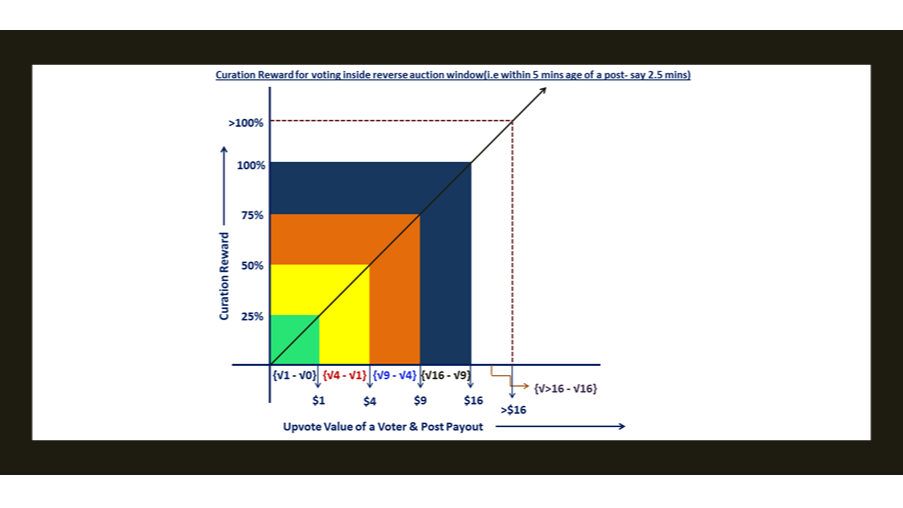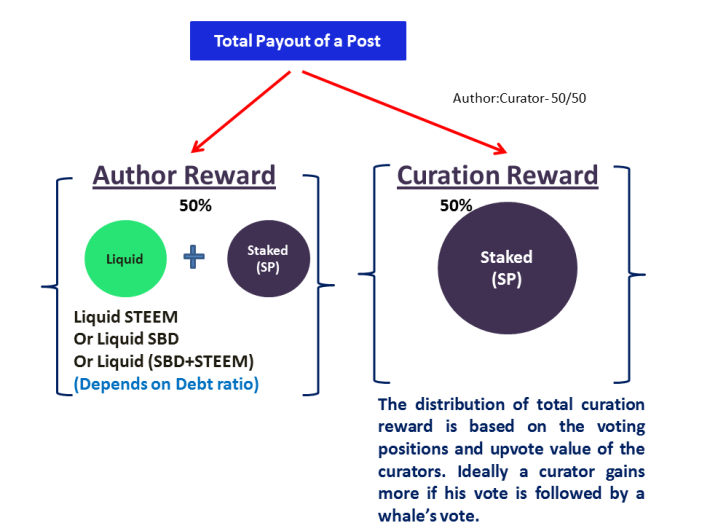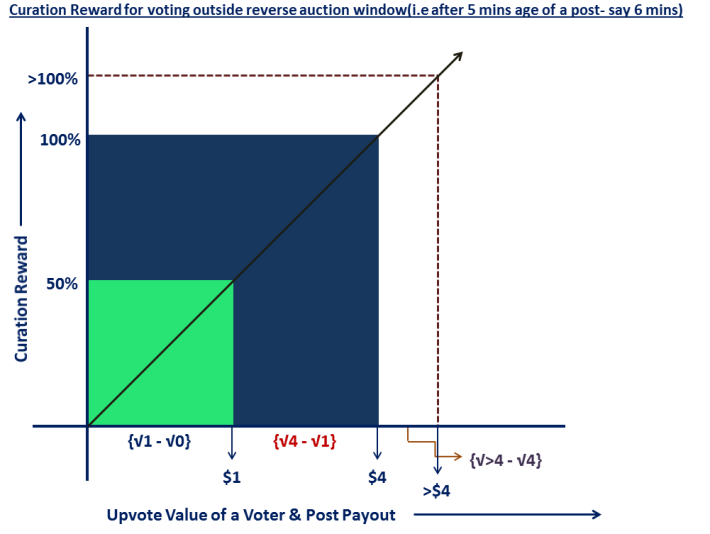Curation Reward in Steem- A curator can earn 100% of the upvote value(or even more) as curation reward with proper strategyCuration Reward in Steem- A curator can earn 100% of the upvote value(or even more) as curation reward with proper strategy

By milaan | scribe | 27 Dec 2019

In Steem, it is possible to get 100% of the upvote value as curation reward or even more.

It is still possible to get 100% of the upvote value as curation reward(or even more) if you vote inside the reverse auction window.

The reverse auction window in Steem is 5 mins inside which if a voter upvotes, then he loses a proportion of his curation reward(which linearly varies from 0 to 5 mins).

A post payout is determined on the basis of proof-of-brain in Steem blockchain where the users of the community determine the post value in a decentralized way by exercising their Steem Power(staked STEEM).

The Author reward and the Curator reward share the post payout in a ratio of 50/50. So the Author gets 50% of the post payout and the Curators get 50% of the post payout. But the curator payout and its distribution is little tricky in Steem blockchain. If a curator properly strategises the voting position, then he can earn 100% of the upvote value or possibly even more if the posts become a popular one and gets attention of the community.I will analyze it in detail with examples. For that let's have a look at the curation reward formula first.

PT= Total Post Payout
P0= Post Payout before voting
P1= Post Payout after voting

The various conditions in regard to curation reward are:

• The author and curator reward split is 50/50. So both of them will get 50% of the total payout.
• The reverse auction window is 5 mins, so voting within this window will result in giving up a part of your curation reward(which varies linearly from 0 to 5 mins) and that will go back to the reward pool. So if you vote at 2.5 mins, you will give up 50% of your curation reward.
• The distribution of curator reward (among the curators) is based on the voting position and upvote value of a curator.

So now we can analyze it.

Assumption- All the voters are voting exactly at the same time. You must remember that the early voters get more curation rewards if a whale votes after them. That is why for simplification purpose this assumption is made that all the voters are voting at the same time.

• Let's say P0=0 and a voter adds \$1 to the post, then P1= 1
• When the next voter comes in & upvote, P0=1, and let's say after the vote the post payout is \$4, then P1=4
• For the next voter P0=4, and let's say after the vote, the post payout is \$9, then P1=9
• For the next voter P0=9, and let's say after the vote, the post payout is \$16, then P1=16

So if we consider that all have voted after the reverse auction window, the payout of the curator will be:

First voter: Pcurator= (1/2) * (√16) * (√1-√0)= 2
Second voter: Pcurator= (1/2) * (√16) * (√4-√1)= 2
Third voter: Pcurator= (1/2) * (√16) * (√9-√4)= 2
Fourth voter: Pcurator= (1/2) * (√16) * (√16-√9)= 2

So if the total post payout is \$16, then total curation reward is \$8(50%) and in the above case if they vote outside the reverse auction window, then they all are getting \$2 each, means they are getting 50% of their upvote value as curation reward(in general the curation reward is 50% of the upvote value)

The reverse auction window is 5 mins.

Now suppose all these four voters vote at 2.5 mins age of the post(i.e. inside the reverse auction window), then Δt= t/5= 2.5/5= 0.5, so the payout of the curator will be:

First voter: Pcurator= (1/2) * (√16) * (√1-√0) * 0.5= 1
Second voter: Pcurator= (1/2) * (√16) * (√4-√1) * 0.5= 1
Third voter: Pcurator= (1/2) * (√16) * (√9-√4) * 0.5= 1
Fourth voter: Pcurator= (1/2) * (√16) * (√16-√9) * 0.5= 1

So if the total post payout is \$16, then total curation reward is \$8 and in the above case if they vote inside the reverse auction window(here it is at 2.5 mins), then they all are getting \$1 each, means they are getting 25% of their upvote value as curation reward.If a voter is voting outside the auction window-- For a post payout of \$4, the initial \$1 voter will get 100% of their upvote value as curation reward. The curation reward can be more than 100% of the upvote value if the post payout is more than \$4 under such a condition.

If a voter is voting inside the auction window-- For a post payout of \$16, voting at 2.5 mins, the initial \$1 voter will get 100% of their upvote value as curation reward. The curation reward can be more than 100% of the upvote value if the post payout is more than \$16 under such a condition.

Simply put, if a voter is voting inside the reverse auction window (say at 2.5 mins), in order to get 100% upvote value as curator reward, the total payout has to be as bigger as 4 times the total payout if the same voter votes outside the reverse auction window(with all other conditions remains same).

The proportions who can potentially make 100% of the upvote value as curation reward is 25% of the total curators. That is really impressive in 50/50 split. Earlier this proportional was 6.25% of the total voters potentially earning 100% of their upvote value as curation reward. So after HF22 and with 50/50 reward split between author and curator as opposed to 75/25 reward split, the potential curation reward has improved 4 times better than 75/25 reward split and that is the reason why most of the people are now engaged in curation in Steem.

Now the curation efficiency has improved and even if you vote inside the reverse auction window you can still get 100% of the upvote value as curation reward and probably more in case of a trending & popular post.

If you are voting at 2.5 mins and if you are among the first \$1 voter, then you can still earn 100% of the upvote value if the total post payout is \$16 or more. You can even earn more than 100% of your upvote value as curation reward in this case if the post payout further goes up beyond \$16.

#### Summary

The total curation reward is 50% of the total post payout. A curator can get "less", "equal to" and "more than 50% of his upvote value" as curation reward subject to the condition that "what is his individual upvote worth" and "what is his upvote position in a particular post" & "what is the total payout of that post". Those who votes later usually get less curation reward than those who vote early but that all depends on the follow through upvote by a whale which potentially makes a post rally in the trending page and hence gain more popularity, attention & reward.

Important NotePlease do note that the actual curation reward will vary & will be more dynamic as it is more sensitive to the timing of the vote, trending & popularity, convergent linear reward, upvote size from whales, etc. The above analysis is simplified based on the assumptions so as to make a better understanding on curation reward for the voters.

The infographics and the analysis are my original works.

Resourcesmilaan

A Blockchain enthusiast, pro-crypto, learning the skills of writing and analyzing blockchain projectsscribe

This blog is about analyzing blockchain technologies and their evolution; reviewing various dApps on the top of blockchain technology and the use-cases they cater to.Send a \$0.01 microtip in crypto to the author, and earn yourself as you read!

20% to author / 80% to me.
We pay the tips from our rewards pool.